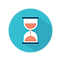top of page

From: Bayesian Models for Astrophysical Data, Cambridge Univ. Press

(c) 2017,  Joseph M. Hilbe, Rafael S. de Souza and Emille E. O. Ishida

you are kindly asked to include the complete citation if you used this material in a publication

Code 4.2  Normal linear model in R using JAGS and the zero trick
==================================================

require(R2jags)

set.seed(1056)                                                   # set seed to replicate example
nobs = 5000                                                      # number of obs in model

x1 <- runif(nobs)                                              # predictor, random uniform variable
beta0 = 2.0                                                        # intercept
beta1 = 3.0                                                        # predictor
xb <- beta0 + beta1 * x1                                  # linear predictor, xb
y <- rnorm(nobs, xb, sd=1)                              # y as an adjusted random normal variate

# Model setup
X <- model.matrix(~ 1 + x1)
K <- ncol(X)

model.data <- list(Y = y,                                 # Response variable
X = X,                               # Predictors
K = K,                               # Number of predictors including the intercept
N = nobs,                          # Sample size
Zeros = rep(0, nobs)         # Zero trick
)

NORM0 <- "
model{

# Diffuse normal priors for predictors
for (i in 1:K) { beta[i] ~ dnorm(0, 0.0001) }

# Uniform prior for standard deviation
tau <- pow(sigma, -2)                                         # precision
sigma ~ dunif(0, 100)                                         # standard deviation

# Likelihood function
C <- 10000

for (i in 1:N){
Zeros[i] ~ dpois(Zeros.mean[i])
Zeros.mean[i] <- -L[i] + C
l1[i] <- -0.5 * log(2*3.1416) - 0.5 * log(sigma)
l2[i] <- -0.5 * pow(Y[i] - mu[i],2)/sigma
L[i] <- l1[i] + l2[i]
mu[i] <- eta[i]
eta[i] <- inprod(beta[], X[i,])
}
}"

inits <- function () {
list(beta = rnorm(K, 0, 0.01))
}

params <- c("beta", "sigma")

norm0fit <- jags(data = model.data,
inits = inits,
parameters = params,
model = textConnection(NORM0),
n.chains = 3,
n.iter = 15000,
n.thin = 1,
n.burnin = 10000)

print(norm0fit,intervals=c(0.025, 0.975), digits=2)Output on screen:

Inference for Bugs model at "3", fit using jags,

3 chains, each with 15000 iterations (first 10000 discarded)

n.sims = 15000 iterations saved

mu.vect         sd.vect                    2.5%                  97.5%         Rhat         n.eff

beta                      1.99              0.03                     1.94                     2.05                1        3500

beta                      3.01              0.05                     2.91                     3.11                1        3600

sigma                       1.00               0.02                     0.96                     1.04                1      15000

deviance   100014175.75              2.49     100014172.93      100014182.29               1              1

For each parameter, n.eff is a crude measure of effective sample size,

and Rhat is the potential scale reduction factor (at convergence, Rhat=1).

DIC info (using the rule, pD = var(deviance)/2)

pD = 3.1 and DIC = 100014178.9

DIC is an estimate of expected predictive error (lower deviance is better).

bottom of page